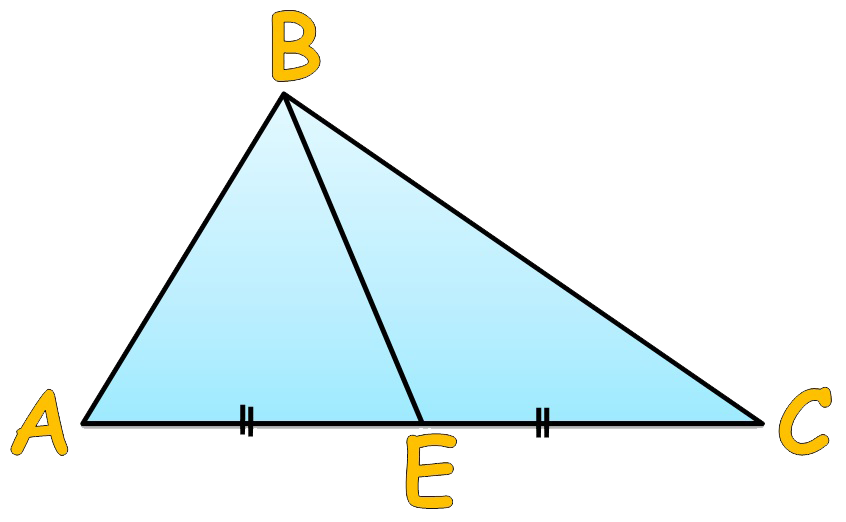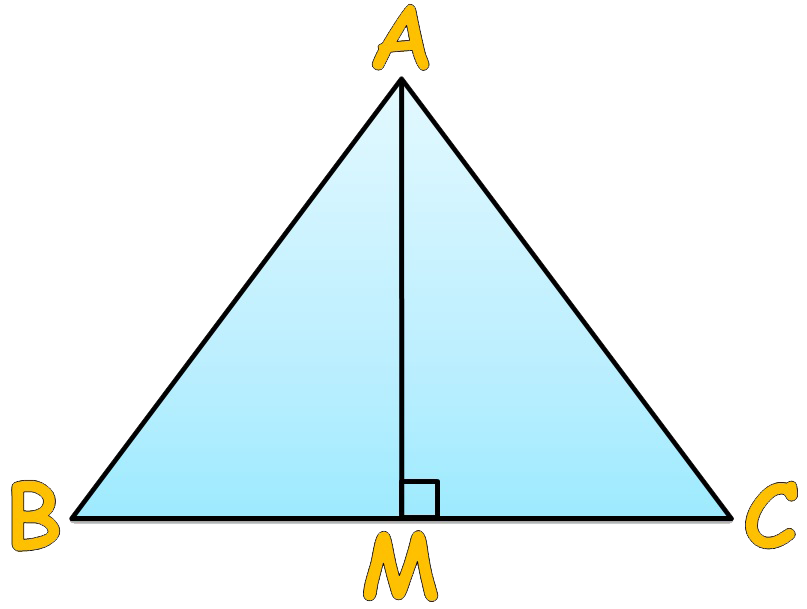# vs.eyeandcontacts.com

## Chapter 6 Triangle and its Properties Exercise 6.1

Question 1: In Δ PQR, D is the mid-point of QR.(i) PM is ____.
PM is an altitude because it has one end point at a vertex of the triangle and other on the line containing the opposite side.

(ii) PD is ____.
PD is a median because a median connects a vertex of a triangle to the mid-point of the opposite side.

(iii) Is QM = MR?
No, QM ≠ MR because M is not the median (which divides the base line segment into equal 2 halves). So QM ≠ MR.

Question 2: Draw rough sketches for the following:
(a) In ΔABC, BE is a median.(b) In ΔPQR, PQ and PR are altitudes of the triangle.(c) In ΔXYZ, YL is an altitude in the exterior of the triangle.